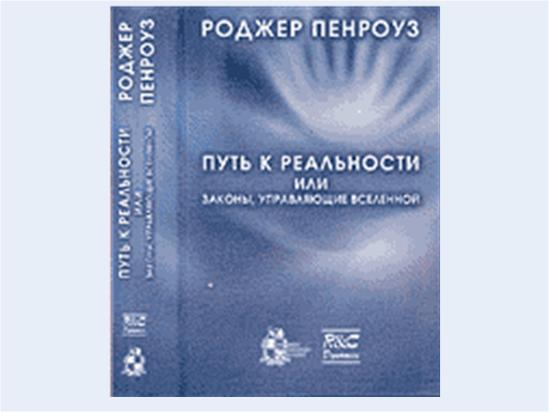Center for Strategic Assessment and forecasts

Autonomous non-profit organization

Home / Science and Society / New in Science / Recommended
R. Penrose `the Road to reality, or the laws that govern the Universe. A comprehensive guide`Material posted: Publication date: 27-02-2011

The purpose of the monograph known physics and mathematics Roger Penrose is the search for fundamental principles underlying our universe, and governing the proceeding processes. We can say that this book is essentially devoted to the relations between mathematics and physics, the interaction between the two disciplines, which plays a significant role in the quest to move on in search of a better theory to describe the Universe.

Specialist in any field will find in this monograph is useful; perhaps the author's point of view on some subjects is different (sometimes quite radically) from the generally accepted, but this will allow us to look at actual problems of modern science from different angles and be closer to the truth. Of great interest is his opinion on several contemporary theories, such as supersymmetry theory, expanding Universe cosmology, hypothesis on the nature of the Big Bang and black holes, string theory or M-theory, loop variables in quantum gravity, the theory of twistors, and in fact the fundamental principles of quantum theory. The book will be of undoubted interest both among the experts of natural-scientific disciplines, and a wide range of readers.

2007. Hardcover. 912 S. 2118 RUB.
ISBN 978-5-93972-618-4

 The content

Preface

Thanks

Prologue

Chapter 1. The origins of science

Chapter 2. An ancient theorem and a modern question

Chapter 3. Types of numbers in the physical world

Chapter 4. Magical complex numbers

Chapter 5. Geometry of logarithms, powers and roots

Chapter 6. Calculus real numbers

Chapter 7. Calculus of complex numbers

Chapter 8. Riemann surfaces and complex mapping

Chapter 9. The Fourier decomposition and hyperfunctions

Chapter 10. The surface

Chapter 11. Hypercomplex functions

Chapter 12. n-dimensional manifolds

Chapter 13. Symmetry groups

Chapter 14. Mathematical analysis on manifolds

Chapter 15. Stratified spaces and gauge connection

Chapter 16. The ladder of infinity

Chapter 17. Space - time

Chapter 18. The Geometry Of Minkowski

Chapter 19. Classical fields of Maxwell and Einstein

Chapter 20. Lagrangians and Hamiltonians

Chapter 21. Quantum particle

Chapter 22. Quantum algebra, geometry and spin

Chapter 23. Entangled quantum world

Chapter 24. Dirac's electron and antiparticles

Chapter 25. Elementary particle physics: standard model

Chapter 26. Quantum field theory

Chapter 27. The big Bang and its thermodynamic legacy

Chapter 28. Speculative theories of the early Universe

Chapter 29. The paradox of measurement

Chapter 30. The role of gravity in quantum state reduction

Chapter 31. Supersymmetry, strings and adrasmansky

Chapter 32. A narrow path of Einstein. Loop variables

Chapter 33. A more radical look. Theory Victorov

Chapter 34. Where lies the path to reality?

Epilogue

Literature

Index

The book is available in the online store:RELATED MATERIALS: Science and Society